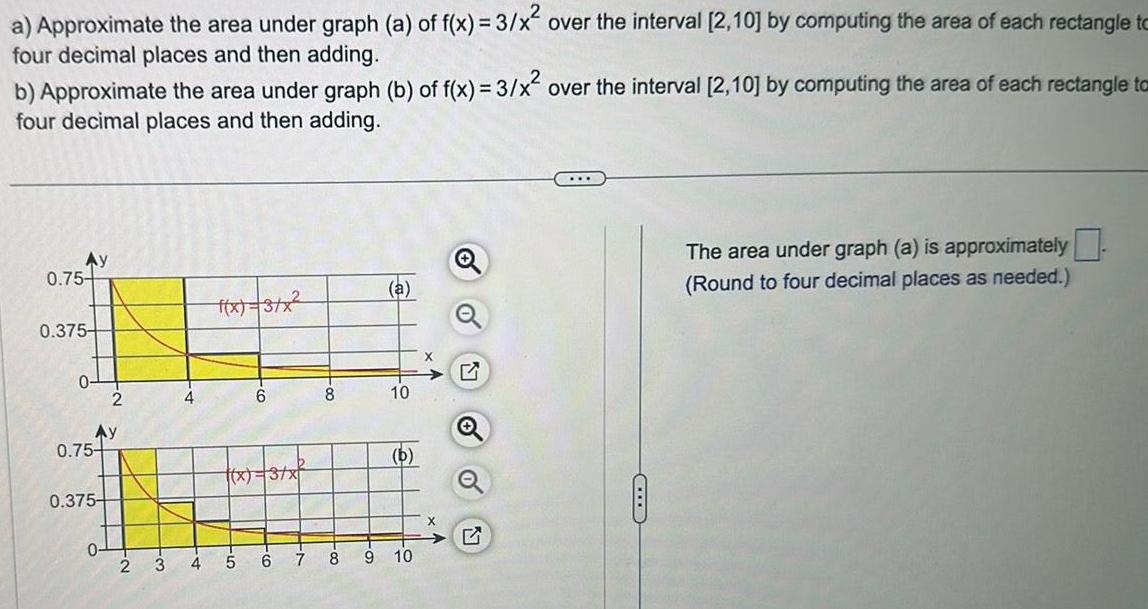Question:

# a Approximate the area under graph a of f x 3 x over the

Last updated: 11/18/2023a Approximate the area under graph a of f x 3 x over the interval 2 10 by computing the area of each rectangle to four decimal places and then adding b Approximate the area under graph b of f x 3 x over the interval 2 10 by computing the area of each rectangle to four decimal places and then adding Ay 0 75 0 375 C 0 Ay 0 75 0 375 2 0 4 4 f x 3 x CO 5 6 x 3 x 6 00 8 a 10 b 7 8 9 10 X O o The area under graph a is approximately Round to four decimal places as needed